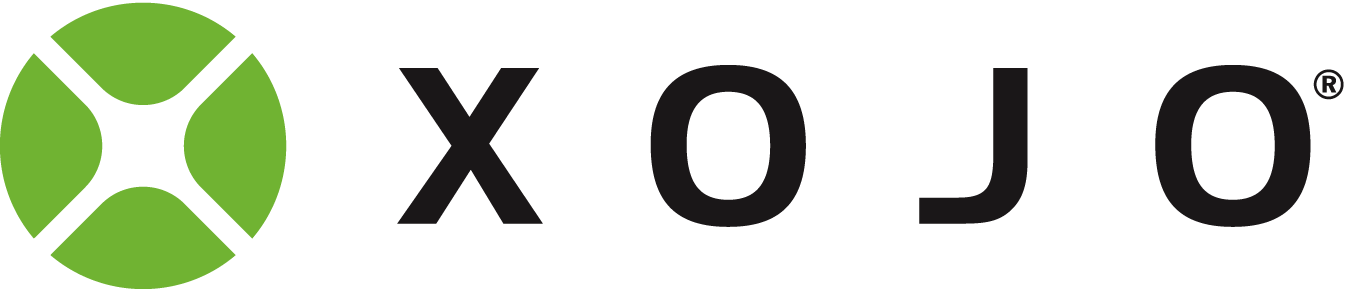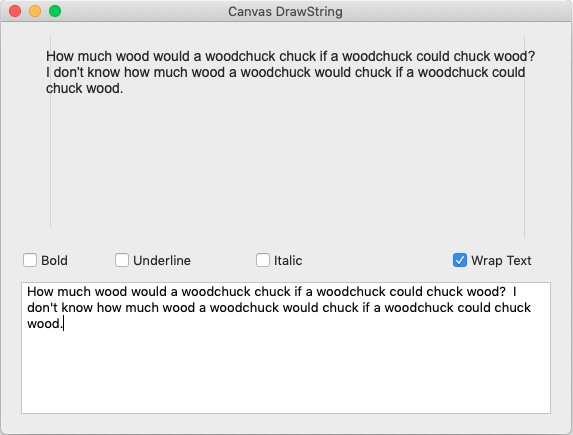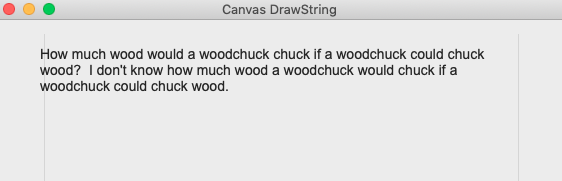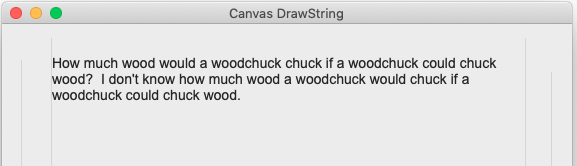How to fit DrawRectangle around DrawText

I would like to know if there is a simple process for DrawText-ing inside of DrawRectangle but Really fit DrawRectangle around DrawText.
I have the process for finding the font size to do this, but my thinking is telling me I’m missing something. Probably related to my inability to understand algebra.
I feel like I’m guessing with getting the locations for the rectangle.

This is how I would do it.

dim padding as integer = 5

dim x as integer = 20
dim y as integer = 20
dim s as string = "Hello World"

g.TextFont = "Arial"
g.TextSize = 14

dim x1 as integer = x - padding
dim y1 as integer = y - g.TextAscent - padding
dim x2 as integer = g.StringWidth(s) + (padding * 2)
dim y2 as integer = g.StringHeight(s, g.width) + (padding * 2)

g.DrawString(s, x, y)
g.DrawRect(x1, y1, x2, y2)
1 Like

I did this too quickly, so the variables are not named very well.
x2 should be w (width)
y2 should be h (height)

g.DrawRect(x1, y1, w, h)

So simple. I was making it much too complicated.

What would change if the text were like this

Lorem ipsum dolor sit amet, consectetur adipiscing elit, sed do eiusmod tempor incididunt ut labore et dolore magna aliqua. Ut enim ad minim veniam, quis nostrud exercitation ullamco laboris nisi ut aliquip ex ea commodo consequat. Duis aute irure dolor in reprehenderit in voluptate velit esse cillum dolore eu fugiat nulla pariatur. Excepteur sint occaecat cupidatat non proident, sunt in culpa qui officia deserunt mollit anim id est laborum.

It worked sort of - enough to learn from. I used the Canvas Draw String example and got 3 sides. The right side was off the edge.

You would have to limit your printable area’s width.

dim leftmargin as integer = 20
dim rightmargin as integer = 20
dim printwidth as integer = g.width - leftmargin - rightmargin

w = min(printwidth, g.StringWidth(s)) + (padding * 2)
h = g.StringHeight(s, printwidth) + (padding * 2)

g.DrawString(s)
g.DrawString(x1, y1, w, h)

Thank you
I’ll post the code for Canvas Drawstring when I’m done playing with it.

I have this piece of text which is going outside of the rectangle and therefore WrapWidth.
Any suggest6ions?

You have probably missed off subtracting a value like the x offset from the DrawString. Post the project if you want to have someon itI left off the wrapwidth
g.DrawString(s, x, y, printwidth)

This is code as it stands:

Const kMargin = 20
Var w As Integer//width
Var h As Integer//height
Var padding as integer = 5
Var leftmargin as integer = 20
Var topmargin As Integer = 20
Var y1 as integer = topmargin - g.FontAscent - padding
Var rightmargin as integer = -20
Var printwidth as integer = g.width - leftmargin - Abs(rightmargin)

// clear the whole canvas
g.ClearRectangle(0, 0, DrawingCanvas.Width, DrawingCanvas.Height)
g.FontName = "Arial"
g.FontSize = 14
g.DrawingColor = Color.TextColor

// set the bold, italic, and underline properties
g.Bold = BoldCheck.Value
g.Underline = UnderlineCheck.Value
g.Italic = ItalicCheck.Value

// draw the string
Var wrapValue As Integer
If WrapCheck.Value Then
wrapValue = Me.Width - kMargin
Else
wrapValue = 0
End If
w = min( printwidth, g.TextWidth(DisplayTextArea.Text) ) + (padding * 2)
h = g.TextHeight(DisplayTextArea.Text, printwidth) + (padding * 2)

g.DrawText(DisplayTextArea.Text, kMargin + padding, kMargin, wrapValue)
g.DrawRectangle( leftmargin, y1, w, h )

Edit:
g.DrawText(DisplayTextArea.Text, kMargin + padding, kMargin, wrapValue)

Should have printwidth instead of wrapWidth.

I am still getting the text outside of the expected boundary. I verified this by adding Lines at 20 pixels in from each side, and the text is 5 outside of it which could be padding is added incorrectly, but I cannot see it.
I also eliminated the rectangle drawing to make it simpler.
What I also cannot verify is the actual computation for DrawText. The code for the paint event is below along with the image.

Const kMargin = 20
Var w As Integer//width
Var h As Integer//height
Var padding as integer = 5
Var leftmargin as integer = 20
Var rightmargin as integer = 20
Var topmargin As Integer = 20
Var y1 as integer = topmargin - g.FontAscent - padding
Var printwidth as integer = g.width - leftmargin - rightmargin

// clear the whole canvas
g.ClearRectangle(0, 0, DrawingCanvas.Width, DrawingCanvas.Height)
g.FontName = "Arial"
g.FontSize = 14
g.DrawingColor = Color.TextColor

// set the bold, italic, and underline properties
g.Bold = BoldCheck.Value
g.Underline = UnderlineCheck.Value
g.Italic = ItalicCheck.Value

// draw the string
Var wrapValue As Integer
If WrapCheck.Value Then
wrapValue = Me.Width - kMargin
Else
wrapValue = 0
End If
'w = min( printwidth, g.TextWidth(DisplayTextArea.Text) + (padding * 2) )
'If printwidth < g.TextWidth(DisplayTextArea.Text)+ (padding * 2) Then
'w = printwidth
'ElseIf printwidth > g.TextWidth(DisplayTextArea.Text) + (padding * 2) Then
'w = g.TextWidth(DisplayTextArea.Text) + (padding * 2)
'End If
'h = g.TextHeight(DisplayTextArea.Text, printwidth) + (padding * 2)

'g.DrawRectangle( leftmargin, y1, w, h )
ReturnArgh. The left side is still too far left and the right is now well very wrongAs above, seems like there’s something going wrong somewhere, probably during the cut and paste.

Thanks Julian
The project is in the examples and its name is Canvas Drawstring.

The only code of any value is what’s above.

I added lines to the Canvas border and changed the Drawtext line to

with this resultThe text on the left is 5 to the left and the right is 20 in.

this code is obviously R3. I’m going to see if 2.1 is any different.

This is what you just posted

This is what I suggested that you try

I’ve no idea where all that extra stuff came from.Cut and paste this whole line ^^^ into your code and hew that other code off into next weekIf it doesn’t work, post the project, I’ll alter it and repost it back working, as there’s some out of sync code going on here or somethingHere’s with the correct DrawText[Dropbox - CanvasDrawString R3 Rect.xojo_xml_project - Simplify your life](CanvasDrawString R3 Rect.xojo_xml_project)

I think I put a separator in the wrong spot.

The left separator has a width of 19 so its offset right a little too much. You also might want to lock the right separator to the right side so it moves when the window alters in width.

https://www.dropbox.com/s/p56b6c83d0rmfib/CanvasDrawString%20%20R4%20Rect.xojo_binary_project?dl=1

I haven’t used separators or lines before. Now I know what width of 20 is and it’s not what I thought. I thought it would be a black blob.

I also miss lines

I think that is my problem

Forum for Xojo Programming Language and IDE. Copyright © 2021 Xojo, Inc.